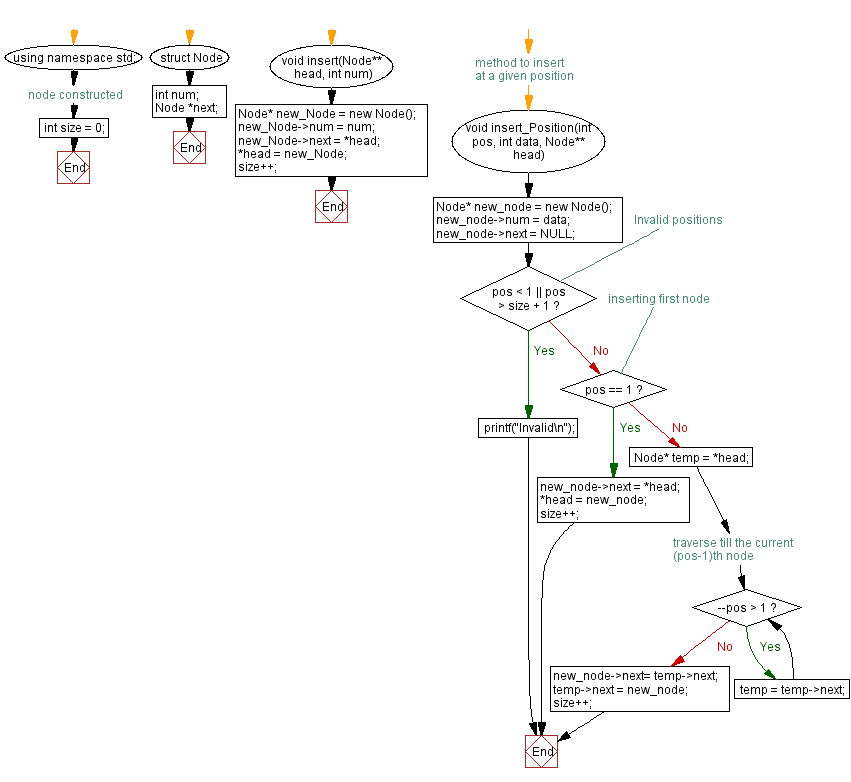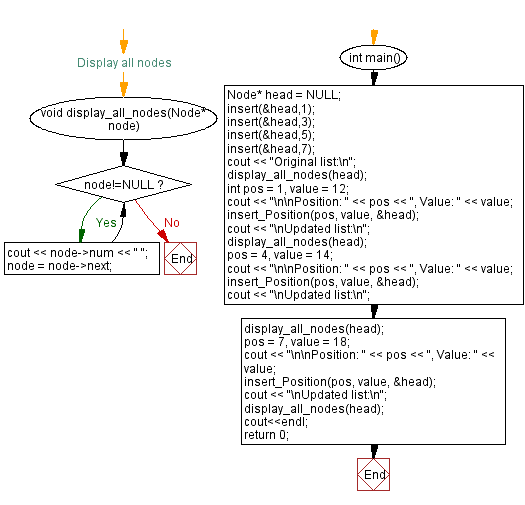﻿ C++ - Insert a node at any position of a Singly Linked List

# C++ Linked List Exercises: Insert a node at any position of a Singly Linked List

## C++ Linked List: Exercise-9 with Solution

Write a C++ program to insert a new node at any position of a Singly Linked List.

Test Data:
Original list:
7 5 3 1
Position: 1, Value: 12
Updated list:
12 7 5 3 1
Position: 4, Value: 14
Updated list:
12 7 5 14 3 1
Position: 7, Value: 18
Updated list:
12 7 5 14 3 1 18

Sample Solution:

C++ Code:

``````#include <iostream>

using namespace std;

struct  Node
{
int num;
Node *next;
}; //node constructed

int size = 0;
Node* new_Node = new Node();
new_Node->num = num;
size++;
}

// method to insert at a given position
void insert_Position(int pos, int data, Node** head)
{
Node* new_node = new Node();
new_node->num = data;
new_node->next = NULL;

// Invalid positions
if(pos < 1 || pos > size + 1)
printf("Invalid\n");

// inserting first node
else if(pos == 1){
size++;
}

else
{

// traverse till the current (pos-1)th node
while(--pos > 1){
temp = temp->next;
}
new_node->next= temp->next;
temp->next = new_node;
size++;
}
}

//Display all nodes
void display_all_nodes(Node* node)
{
while(node!=NULL){
cout << node->num << " ";
node = node->next;
}
}

int main()
{
cout << "Original list:\n";
int pos = 1, value = 12;
cout << "\n\nPosition: " << pos << ", Value: " << value;
cout << "\nUpdated list:\n";
pos = 4, value = 14;
cout << "\n\nPosition: " << pos << ", Value: " << value;
cout << "\nUpdated list:\n";
pos = 7, value = 18;
cout << "\n\nPosition: " << pos << ", Value: " << value;
cout << "\nUpdated list:\n";
cout<<endl;
return 0;
}
``````

Sample Output:

```Original list:
7 5 3 1

Position: 1, Value: 12
Updated list:
12 7 5 3 1

Position: 4, Value: 14
Updated list:
12 7 5 14 3 1

Position: 7, Value: 18
Updated list:
12 7 5 14 3 1 18
```

Flowchart:CPP Code Editor: